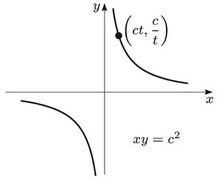×#### Thank you for registering.

One of our academic counsellors will contact you within 1 working day.

Click to Chat

1800-1023-196

+91-120-4616500

CART 0

• 0

MY CART (5)

Use Coupon: CART20 and get 20% off on all online Study Material

ITEM
DETAILS
MRP
DISCOUNT
FINAL PRICE
Total Price: Rs.

There are no items in this cart.
Continue Shopping```Rectangular Hyperbola

What do we mean by a Rectangular Hyperbola?The hyperbola whose asymptotes are at right angles to each other is called a rectangular hyperbola. The angle between asymptotes of the hyperbola x2/a2 – y2/b2 = 1, is 2 tan–1 (b/a).

This is a right angle if tan–1 b/a = π/4, i.e., if b/a = 1 ⇒ b = a.

The equation of rectangular hyperbola referred to its transverse and conjugate axes as axes of coordinates is therefore x2 – y2 = a2.

How do we compute the Rectangular Hyperbola Equation?

We know that the asymptotes of the hyperbola x2/a2 – y2/b2 = 1     …… (1)

are given by y = + (b/a) x                         …… (2)

If θ be the angle between the asymptotes, then

θ = tan–1 ((m1–m2)/(1 + m1m2))

= tan–1 [{(b/a)–(–b/a)}/{1+(b/a)(–b/a)}]

= tan–1 [2(b/a)/(1–(b2/a2))]

= 2 tan–1 (b/a)

But if the hyperbola is rectangular, then θ = π/2

i.e., π/2 = 2 tan–1 (b/a) or tan (π/4) = b/a

⇒ b = a

Therefore, from (1) the equation of the rectangular hyperbola is x2 – y2 = a2.

In order to obtain the equation of the hyperbola which has asymptotes as coordinate axis we rotate the axes of reference through an angle of -45o.

Hence, for this we have to write x/√2 + y/√2 for x and –x/√2 + y/√2 for y.

The equation (i) becomes

(1/2)(x + y)2 – (1/2)(x – y)2 = a2 i.e.

xy = ½ a2 or xy = c2 where c2 = a2/2.Watch this Video for more reference

Some Important Points to be noted:

In a hyperbola b2 = a2 (e2 – 1). In the case of rectangular hyperbola (i.e., when b = a) result becomes a2 = a2(e2 – 1) or e2 = 2 or e = √2

i.e. the eccentricity of a rectangular hyperbola = √2.

In case of rectangular hyperbola a = b i.e., the length of transverse axis = length of conjugate axis.

A rectangular hyperbola is also known as an equilateral hyperbola.

The asymptotes of rectangular hyperbola are y = ± x.

If the axes of the hyperbola are rotated by an angle of -π/4 about the same origin, then the equation of the rectangular hyperbola x2 – y2 = a2 is reduced to xy = a2/2 or xy = c2.

When xy = c2, the asymptotes are the coordinate axis.

Length of latus rectum of rectangular hyperbola is the same as the transverse or conjugate axis.

Rectangular Hyperbola with asymptotes as coordinate axis:

The equation of the hyperbola which has its asymptotes as the coordinate axis is xy = c2 with parametric representation x = ct and y = c/t, t ∈ R-{0}.

The equations of the directrices of the hyperbola in this case are x + y = ± √2c.

Since, the transverse and the conjugate axes are the same hence, length of latus rectum = 2√2c = T.A. = C.A.

Equation of a chord whose middle point is given to be (p, q) is qx + py = 2pq.

The equation of the tangent at the point P(x1, y1) is x/x1 + y/y1 = 2 and at P(t) is x/t + ty = 2c.

Equation of normal is y-c/t = t2(x-ct).

The equation of the chord joining the points P(t1) and Q(t2) is x + t1t2y = c(t1 + t2) and its slope is m = -1/t1t2.

The vertices of the hyperbola are (c, c) and (-c, -c) and the focus is (√2c, √2c) and (-√2c, -√2c).

A rectangular hyperbola circumscribing a triangle passes through the orthocentre of this triangle.

If a circle intersects a rectangular hyperbola at four points, then the mean value of the points of intersection is the mid-point of the line joining the centres of both circle and hyperbola.

Illustration:

Find the equation of the hyperbola with asymptotes 3x – 4y + 9 = 0 and 4x + 3y + 1 = 0 which passes through the origin.

Solution:

The asymptotes are given as 3x – 4y + 7 = 0 and 4x + 3y + 1 = 0.

Hence, the joint equation of the asymptotes is (3x – 4y + 9)(4x + 3y + 1) = 0.

Now, we know that the equation of rectangular hyperbola differs from that of the joint equation of asymptotes by a constant only, hence we must have the equation of the hyperbola as

(3x – 4y + 9)(4x + 3y + 1) + r = 0.

It is given that the hyperbola passes through the origin and hence, putting x = y = 0 , we obtain,

9 + r = 0

Hence, r = -9.

Hence, the equation of the hyperbola is (3x – 4y + 9)(4x + 3y + 1) – 9 = 0.

This gives 12x2 + 9xy + 3x -16xy – 12y2 – 4y + 36x + 27y + 9 – 9 = 0.

So, 12x2 – 12y2 - 7xy + 39x + 23y = 0.

Related Resources

For getting an idea of the type of questions asked, refer the previous year papers.

To read more, Buy study materials of Hyperbola comprising study notes, revision notes, video lectures, previous year solved questions etc. Also browse for more study materials on Mathematics here.
```### Course Features

• 731 Video Lectures
• Revision Notes
• Previous Year Papers
• Mind Map
• Study Planner
• NCERT Solutions
• Discussion Forum
• Test paper with Video Solution